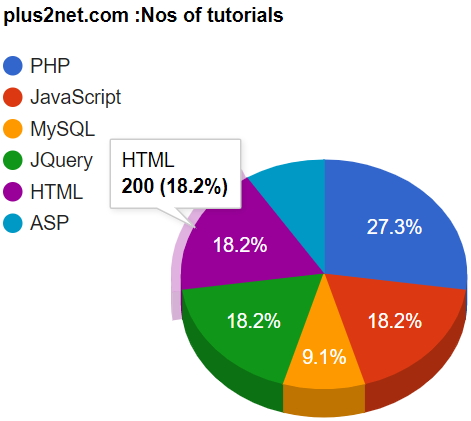# Pie Chart using data from MySQL table by PHPFile NameDetails
config.php MySQLi Database connection details are stored here.
sql_dump.txt SQL Dump to create chart_data table with sample data.
readme.txt Instructions on how to run the script
index.php The main file to display records and the Pie chart.
Using PDO : PHP Data Object
File NameDetails
config-pdo.php PDO Database connection details are stored here.
index-pdo.php Using PDO the main file to display Pie Chart.
Using CSV (Comma Separated Value) file :
File NameDetails
index-csv.php Reading CSV file by using fgetcsv() and display chart.
chart_data.csv Comma separated value ( csv ) file with data.

Pie Chart with data from MySQL database table using PHP pdo and google chart library

## 1 : Collecting data from MySQL database

After connecting to database through config.php file, we will run the SQL to collect the data from MySQL table. You can read more on MySQLi and how to collect data from table.
``````if(\$stmt = \$connection->query("SELECT language, nos  FROM chart_data")){

echo "No of records : ".\$stmt->num_rows."<br>";
\$php_data_array = Array(); // create PHP array
echo "<table>
<tr> <th>Language</th><th>Nos</th></tr>";
while (\$row = \$stmt->fetch_row()) {
echo "<tr><td>\$row</td><td>\$row</td></tr>";
\$php_data_array[] = \$row; // Adding to array
}
echo "</table>";
}else{
echo \$connection->error;
}``````
Using PDO : PHP Data Object we can connect to MySQL and retrive the data to create the array \$php_data_arra.
``````<?Php
require "config-pdo.php";// Database connection

\$query="SELECT language, nos  FROM chart_data";
\$step = \$dbo->prepare(\$query);
if(\$step->execute()){
\$php_data_array = \$step->fetchAll();
//echo json_encode(\$php_data_array);

//Transfor PHP array to JavaScript two dimensional array
echo "<script>
var my_2d = ".json_encode(\$php_data_array)."
</script>";
}else{
echo \$connection->error;
}
?>``````

## 2 : Data array in PHP

Before displaying records in a table we have created an array in PHP ( `\$php_data_array`). While displaying the records in a table we store each record inside the PHP array.
``\$php_data_array[] = \$row; // Adding to array``
After displaying all the records in a table we have the `\$php_data_array` with all the data collected from MySQL table. We can display the Json string like this.
``echo json_encode(\$php_data_array);``

## 3 : Transferring data from PHP to JavaScript to crate the chart

By using json_encode we will create an json string which can be used to create the JavaScript two dimensional array.
``````echo "<script>
var my_2d = ".json_encode(\$php_data_array)."
</script>";``````

## 4 : Adding data to Chart

Our JavaScript array `my_2d` stores all the data required for creating the chart. We need to display them in the format required by our Chart library.
``````for(i = 0; i < my_2d.length; i++)
We used parseInt() function to convert input string value to Integer. JavaScript part is here .
``````<script type="text/javascript" src="https://www.gstatic.com/charts/loader.js"></script>
<script>
// Draw the pie chart when Charts is loaded.
// Callback that draws the pie chart
function draw_my_chart() {
// Create the data table .
for(i = 0; i < my_2d.length; i++)
// above row adds the JavaScript two dimensional array data into required chart format
var options = {title:'plus2net.com : How the tutorials are distributed',
width:600,
height:500};

// Instantiate and draw the chart
chart.draw(data, options);
}
</script>``````

## 5. HTML part

We can show the chart inside a <DIV> tag and place the same any where we want to display the chart.
``<div id="chart_div"></div>``

The chart drawn above can be displayed with live internet connection as the library is to be loaded from gstatic.com

Data is collected from MySQL database table so data can be edited by using script and the same will be reflected by the Chart. We have not included this part in the present script and there are many tutorials available here one handling data using PHP and MySQL.

You can read tutorials on other types of charts displayed by using data from database.

## Changing Height Width and Title

``````var options = {'title':'Tutorials at plus2net.com',
'width':400,
'height':300};``````

## 3-D Pie chart

Change the options and add `is3D=true`, by default its value is false.
``````var options = {title:'plus2net.com :Nos of tutorials',
width:600,
height:500,
legend:'left',
is3D:true};``````## Using CSV file as data source

Once the php aray `\$php_data_array` is created the rest of the code remain same. Here we are reading the chart_data.csv file to create the `\$php_data_array`. Once the array is created same code can be used. Zip file below contains the chart_data.csv file as data source and the index-csv.php file to display the chart.
We used fgetcsv() to read csv file
``````\$f_pointer=fopen("chart_data.csv","r"); // file pointer
\$php_data_array = Array(); // create PHP array
while(! feof(\$f_pointer)){
\$ar=fgetcsv(\$f_pointer);
//echo print_r(\$ar); // print the array
\$php_data_array[] = \$ar; // Adding to array
}
//print_r(\$php_data_array);
echo "<script>
var my_2d=".json_encode(\$php_data_array)."
</script>";``````Subscribe to our YouTube Channel here

## Subscribe

* indicates required
Subscribe to plus2netplus2net.com